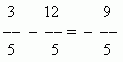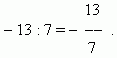# Rational numbers

Negative integers. Series of negative integers.
Fractional negative numbers. Positive numbers.
Rational numbers.

Negative integers appear, when the greater integer is subtracted from the smaller one, for instance:

10 – 15 = – 5 .

The sign “minus” before  5  shows, that this number is negative.

Series of negative integers continue endlessly:

–1,   –2,  –3,  – 4,  –5, …

Integers are natural numbers, negative integers and zero:

... ,  –3,  –2,  –1,  0,  1,  2,  3, ...

Fractional negative numbers appear, for example, when the greater number is subtracted from the smaller one:Also it is possible to say, that fractional negative numbers appear as a result division of a negative integer by a natural number:Positive numbers in contrast to negative numbers (integers and fractional ones), are the numbers, considered in arithmetic (also integers and fractional ones).

Rational numbers – positive and negative numbers (integers and fractional ones) and zero. The more exact definition of rational numbers, adopted in mathematics, is the following:

A number is called rational , if it may be presented as a vulgar, not a cancelled fraction of the shape: m / n , where m is an integer, and n is a natural number.